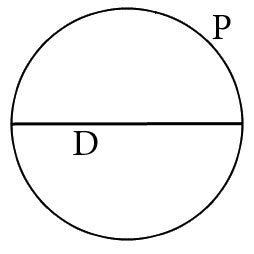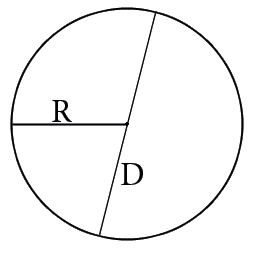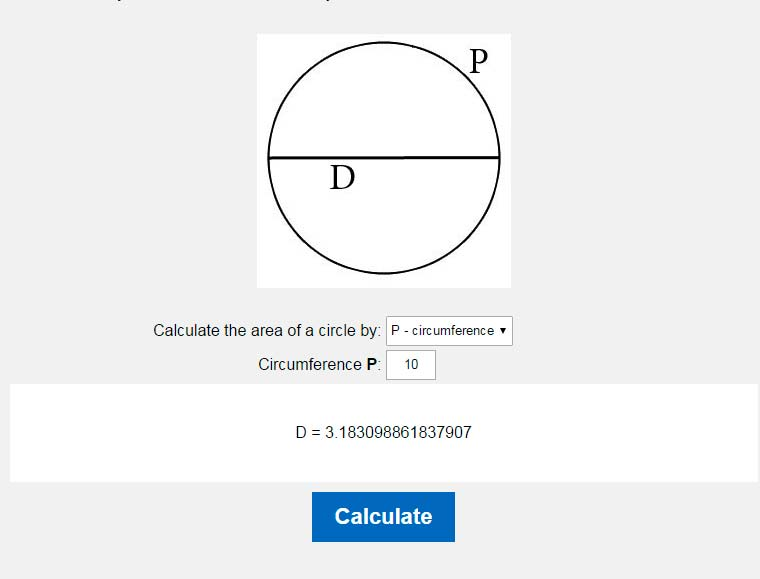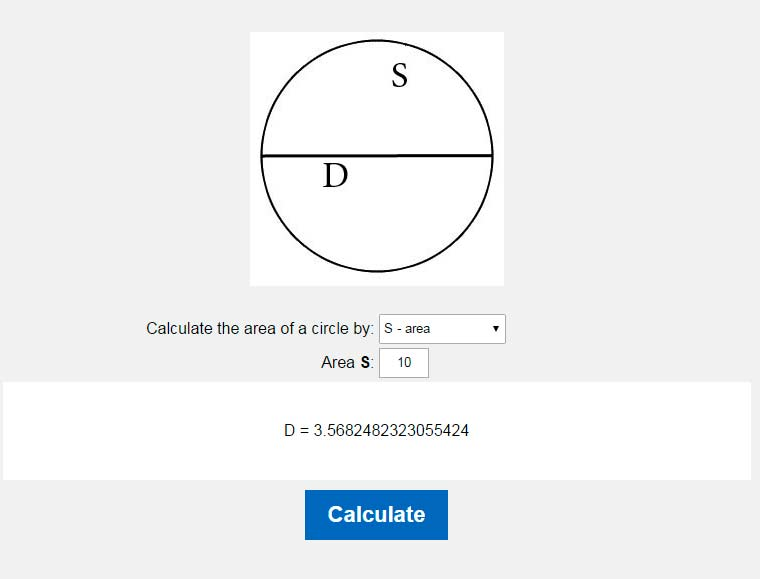# Diameter of a circle calculator. Calculate the diameter of a circle.

You could easy calculate circle's diameter by our online calculator.Calculate the area of a circle by: P - circumferenceS - areaR - radius Circumference P:

In order to calculate the diameter of the circle must know the length or area. If we know one of the ukazannh values will not be difficult to calculate the diameter of the circle for us.
The diameter of the circle is calculated using the following formulas:

1. If we know the length:
The formula for calculating the diameter of the circle through its length:
D=P/π2. If we know the area:
The formula for calculating the diameter of the circle through the area:
D=2S/π3. If we know the diameter:
The formula for calculating the diameter of the circle of the radius:
D=2RWhere D - diameter of the circle, S - area of a circle, P - the length of the circle, R - radius, π - Pi is always approximately equal to 3.14.

### The diameter of the circle through its length### The diameter of the circle through the area### The diameter of the circle through the radiusHOME
RESOURCES